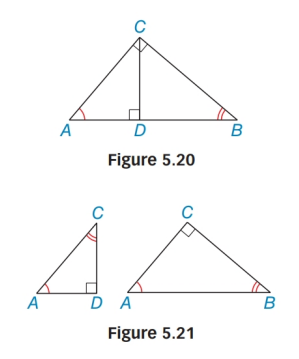Chapter 5.4, Problem 44E### Elementary Geometry for College St...

6th Edition
Daniel C. Alexander + 1 other
ISBN: 9781285195698

#### Solutions

Chapter
Section### Elementary Geometry for College St...

6th Edition
Daniel C. Alexander + 1 other
ISBN: 9781285195698
Textbook Problem
20 views

# Use Figures 5.20 and 5.21 to prove Lemma 5.4.3.Lemma 5.4.3The length of each leg of a right triangle is the geometric mean of the length of the hypotenuse and the length of the segment of the hypotenuse adjacent to that leg.

To determine

To prove :

The lemma 5.4.3 “The length of each leg of a right triangle is the geometric mean of the length of the hypotenuse and the length of the segment of the hypotenuse adjacent to that leg”.

Explanation

Given figures 5.19 and 5.20

From the figure ΔABC, with right ACB; CD¯AB¯

Now we have to prove ABAC=ACAD.

By forming a table,

 1 ΔABC with right ∠ACBCD¯⊥AB¯ Given 2 CD¯ is an altitude to AB¯ AN altitude is a segment from a vertex and perpendicular the opposite side

### Still sussing out bartleby?

Check out a sample textbook solution.

See a sample solution

#### The Solution to Your Study Problems

Bartleby provides explanations to thousands of textbook problems written by our experts, many with advanced degrees!

Get Started

#### Find more solutions based on key concepts